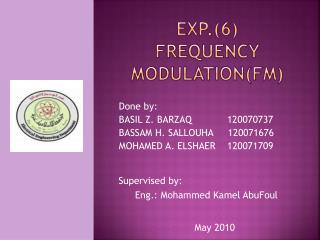Download PresentationExp.(6) Frequency modulation(fm)

Loading in 2 Seconds...

# Exp.(6) Frequency modulation(fm) - PowerPoint PPT Presentation

Exp.(6) Frequency modulation(fm). Done by: BASIL Z. BARZAQ 120070737 BASSAM H. SALLOUHA 120071676 MOHAMED A. ELSHAER 120071709. Supervised by: Eng.: Mohammed Kamel AbuFoul. May 2010. OBJECTIVES.I am the owner, or an agent authorized to act on behalf of the owner, of the copyrighted work described.
Download Presentation## Exp.(6) Frequency modulation(fm)

An Image/Link below is provided (as is) to download presentation

Download Policy: Content on the Website is provided to you AS IS for your information and personal use and may not be sold / licensed / shared on other websites without getting consent from its author.While downloading, if for some reason you are not able to download a presentation, the publisher may have deleted the file from their server.

- - - - - - - - - - - - - - - - - - - - - - - - - - E N D - - - - - - - - - - - - - - - - - - - - - - - - - -
Presentation Transcript
1. Exp.(6)Frequency modulation(fm) Done by: BASIL Z. BARZAQ 120070737 BASSAM H. SALLOUHA 120071676 MOHAMED A. ELSHAER 120071709 Supervised by: Eng.: Mohammed Kamel AbuFoul May 2010

2. OBJECTIVES • Understanding frequency modulation and demodulation by direct differentiation ,slope detection. • Using MATLAB m-file and simulink to implement FM modulation ,demodulation.

3. Frequency modulation • Definition: it’s a form of modulation which represents information as variations in the instantaneous frequency of a carrier wave. • Note: 1)In analog applications,the carrier frequency is varied in direct proportion to changes in the amplitude of an input signal.

4. 2) The FM-modulated signal has its instantaneous frequency that varies linearly with the amplitude of the message signal. Now we can get the FM-modulation by: Where: Kƒ is the sensitivity factor, and represents the frequency deviation rate as a result of message amplitude change. The instantaneous frequency is:

5. The maximum deviation of Fc (which represents the max. shift away from Fc in one direction) is: Note: The FM-modulation is implemented by controlling the instantaneous freq. of a voltage-controlled oscillator(VCO). The amplitude of the input signal controls the oscillation freq. of the VCO output signal.

6. Fm demodulation • In the FM demodulation what we need to recover is the variation of the instantaneous frequency of the carrier, either above or below the center frequency. • The detecting device must be constructed so that its output amplitude will vary linearly according to the instantaneous freq. of the incoming signal.

7. Fm demodulation by direct differentiation • In this method we differentiate the FM signal to get an AM signal, then we use an envelope detector.

8. Matlab codes • The code of plotting m(t) and FM modulated signal: clear all fc=100; ts=1/(10*fc); fs=(1/ts); kf=80; wc=2*pi*fc; t=0:ts:2; m=sin(2*pi*t); y=cos(wc*t+(kf*2*pi*cumsum(m)).*ts); figure(1) subplot(211) plot(t,m) title('input signal') subplot(212) plot(t,y) title('fm modulation of input signal')

9. The code of magnitude spectrum of m(t) and the FM signal: mf=fftshift(fft(m))*ts; delta=fs/length(mf); f=-fs/2:delta:fs/2-delta; figure(2) subplot(211) plot(f,abs(mf)) title('magnitude spectrum of input signal') a=fftshift(fft(y))*ts; delta=fs/length(a); f=-fs/2:delta:fs/2-delta; subplot(212) plot(f,abs(a)) title('magnitude spectrum of the fm')

10. The plot of the output signal after differentiator: E=diff(y)/ts; figure(3) plot(E) title('the differentiation of fm ')

11. The plot of the output signal from the envelope detector: vout(1)=E(1); t1=(0:length(E)-1)*ts; R=[10^5,10^4,10^3,10^2]*3.2; c=10^-6; for n=1:4 for i =2:length(E) if E(i)>vout(i-1) vout(i)=E(i); else vout(i)=vout(i-1).*exp(-ts/(R(n)*c)); end end figure(4) subplot(4,1,n) plot(t1,vout,t1,E) title(' the AM signal and envelope signal ') end

12. R= 10^5*3.2

13. R=10^4*3.2

14. R= 10^3*3.2

15. R= 10^2*3.2

16. THANK YOU